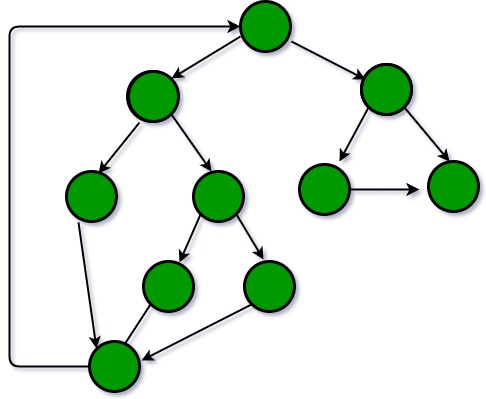# GATE | GATE-CS-2015 (Set 1) | Question 65

Consider the following C program segment.

 `while` `(first <= last) ` `{ ` `   ``if` `(array [middle] < search) ` `      ``first = middle +1; ` `   ``else` `if` `(array [middle] == search) ` `      ``found = True; ` `   ``else` `last = middle – 1; ` `   ``middle = (first + last)/2; ` `} ` `if` `(first < last) not Present = True; `

The cyclomatic complexity of the program segment is __________.

(A) 3
(B) 4
(C) 5
(D) 6

Explanation: the cyclomatic complexity of a structured program[a] is defined with reference to the control flow graph of the program, a directed graph containing the basic blocks of the program, with an edge between two basic blocks if control may pass from the first to the second. The complexity M is then defined as

```
M = E − N + 2P,
where
E = the number of edges of the graph.
N = the number of nodes of the graph.
P = the number of connected components.  ```

For a single program (or subroutine or method), P is always equal to 1. So a simpler formula for a single subroutine is

`    M = E − N + 2 `

For the given program, the control flow graph is:``` E = 13, N = 10.

Therefore, E - N + 2 = 5. ```

My Personal Notes arrow_drop_up

Article Tags :

Be the First to upvote.

Please write to us at contribute@geeksforgeeks.org to report any issue with the above content.